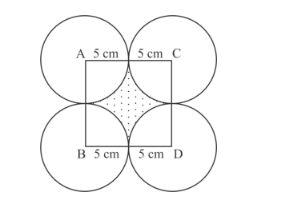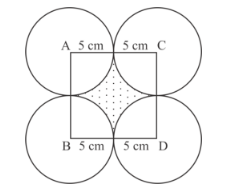# Four equal circles, each of radius 5 cm,Question:

Four equal circles, each of radius 5 cm, touch each other as shown in the following figure. Find the area included between them (Take π = 3.14).Solution:

It is given that four equal circle touches each other as shown in figure.Let the side of square is a.

$a=5+5$

$=10 \mathrm{~cm}$

Area of square $=a^{2}$

$=10 \times 10$

$=100 \mathrm{~cm}^{2}$

We know that

Area of circle of radius $r=\pi r^{2}$

$=3.14 \times 5 \times 5$

$=78.5 \mathrm{~cm}^{2}$

Area of quadrant inside square $=\frac{1}{4} \pi r^{2}$

$=\frac{1}{4} \times 78.5 \mathrm{~cm}^{2}$

Area of shaded region $=$ Area of square $-4 \times$ Area of quadrant

$=100-4 \times \frac{1}{4} \times 78.5$

$=100-78.5$

$=21.5 \mathrm{~cm}^{2}$